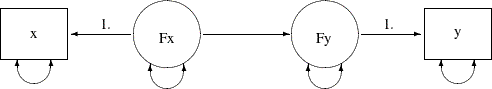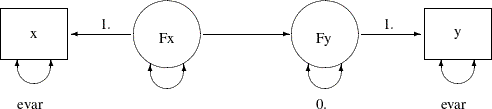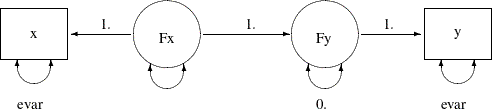# The CALIS Procedure

### Example 29.9 Testing Specific Measurement Error Models

Subsections:

In Example 29.8, you used the PATH modeling language of PROC CALIS to fit some basic measurement error models. In this example, you continue to fit the same kind of measurement error models but you restrict some model parameters to test some specific hypotheses.

This example uses the same data set as is used in Example 29.8. This data set contains 30 observations for the x and y variables. The general measurement error model with measurement errors in both x and y is shown in the following path diagram:

Output 29.9.1:In the path diagram, two paths are fixed with a path coefficient of 1. They are required in the model for representing the relationships between true scores (latent) and measured indicators (observed). In Example 29.8, you consider several different modeling scenarios, all of which require you to make some parameter restrictions to estimate the models. You fix the measurement error variances to certain values that are based on prior knowledge or studies. Without those fixed error variances, those models would have been overparameterized and the parameters would not have been estimable.

For example, in the current path diagram, five of the single- or double-headed paths are not labeled with fixed numbers. Each of these paths represents a free parameter in the model. However, in the covariance structure model analysis, you fit these free parameters to the three nonredundant elements of the sample covariance matrix, which is a 22 symmetric matrix. Hence, to have an identified model, you can at most have three free parameters in your covariance structure model. However, the path diagram shows that you have five free parameters in the model. You must introduce additional parameter constraints to make the model identified.

If you do not have prior knowledge about the measurement error variances (as those described in Example 29.8), then you might need to make some educated guesses about how to restrict the overparameterized model. For example, if x and y are of the same kind of measurements, perhaps you can assume that they have an equal amount of measurement error variance. Furthermore, if the measurement errors have been taken into account, in some physical science studies you might be able to assume that the relationship between the true scores Fx and Fy is almost deterministic, resulting in a near zero error variance of Fy.

The assumptions here are not comparable to prior knowledge or studies about the measurement error variances. If you suppose they are reasonable enough in a particular field, you can use these assumptions to give you an identified model to work with (at least as an exploratory study) when the required prior knowledge is lacking. The following path diagram incorporates these two assumptions in the measurement error model:

Output 29.9.2:In the path diagram, you use evar to denote the error variances of x and y. This implicitly constrains the two error variances to be equal. The error variance of Fy is labeled zero, indicating a fixed parameter value and a deterministic relationship between x and y. You can transcribe this path diagram into the following PATH modeling specification:

proc calis data=measures;
path
x  <=== Fx  = 1.,
Fx ===> Fy   ,
Fy ===> y   = 1.;
pvar
x  = evar,
y  = evar,
Fy = 0.,
Fx;
run;


In the PVAR statement, you specify the same parameter name evar for the error variances of x and y. This way their estimates are constrained to be the same in the estimation. In addition, the error variance for Fy is fixed at zero, which reflects the "near-deterministic" assumption about the relationship between Fx and Fy. These two assumptions effectively reduce the overparameterized model by two parameters so that the new model is just-identified and estimable.

Output 29.9.3 shows the estimation results. The estimated effect of Fx on Fy is 1.3028 (standard error = 0.1134). The measurement error variances for x and y are both estimated at 0.0931 (standard error = 0.0244).

Output 29.9.3: Estimates of the Measurement Error Model with Equal Measurement Error Variances

PATH List
Path Parameter Estimate Standard
Error
t Value Pr > |t|
x <=== Fx   1.00000
Fx ===> Fy _Parm1 1.30275 0.11336 11.4924 <.0001
Fy ===> y   1.00000

Variance Parameters
Variance
Type
Variable Parameter Estimate Standard
Error
t Value Pr > |t|
Error x evar 0.09307 0.02444 3.8079 0.0001
y evar 0.09307 0.02444 3.8079 0.0001
Fy   0
Exogenous Fx _Parm2 0.70655 0.20962 3.3706 0.0008

#### Testing the Effect of Fx on Fy

Suppose you are interested in testing the hypothesis that the effect of Fx on Fy (that is, the regression slope) is 1. The following path diagram represents the model under the hypothesis:

Output 29.9.4:Now you label the path from Fx to Fy with a fixed constant 1, which reflects the hypothesis you want to test. You can transcribe the current path diagram easily into the following PROC CALIS specification:

proc calis data=measures;
path
x  <=== Fx  = 1.,
Fx ===> Fy  = 1.,  /* Testing a fixed constant effect */
Fy ===> y   = 1.;
pvar
x  = evar,
y  = evar,
Fy = 0.,
Fx;
run;


Output 29.9.5 shows the model fit chi-square statistic. The model fit chi-square test here essentially is a test of the null hypothesis of the constant effect at 1 because the alternative hypothesis is a saturated model. The chi-square value is 8.1844 (df = 1, p = .0042), which is statistically significant. This means that the hypothesis of constant effect at 1 is rejected.

Output 29.9.5: Fit Summary for Testing Constant Effect

Fit Summary
Chi-Square 8.1844
Chi-Square DF 1
Pr > Chi-Square 0.0042

Output 29.9.6 shows the estimates under this restricted model. In the first table of Output 29.9.6, all path effects or coefficients are fixed at 1. In the second tale of Output 29.9.6, estimates of the error variances are 0.1255 (standard error = 0.0330) for both x and y. The error variance of Fy is a fixed zero, as required in the hypothesis. The variance estimate of Fx is 0.9205 (standard error = 0.2587).

Output 29.9.6: Estimates of Constant Effect Measurement Error Model

PATH List
Path Estimate Standard
Error
t Value Pr > |t|
x <=== Fx 1.00000
Fx ===> Fy 1.00000
Fy ===> y 1.00000

Variance Parameters
Variance
Type
Variable Parameter Estimate Standard
Error
t Value Pr > |t|
Error x evar 0.12545 0.03295 3.8079 0.0001
y evar 0.12545 0.03295 3.8079 0.0001
Fy   0
Exogenous Fx _Parm1 0.92046 0.25872 3.5577 0.0004

#### Testing a Zero Intercept

Suppose you are interested in testing the hypothesis that the intercept for the regression of Fy on Fx is zero, while the regression effect is freely estimated. Because the intercept parameter belongs to the mean structures, you need to specify this parameter in PROC CALIS to test the hypothesis.

There are two ways to include the mean structure analysis. First, you can include the MEANSTR option in the PROC CALIS statement. Alternatively, you can use the MEAN statement to specify the means and intercepts in the model. The following statements specify the model under the zero intercept hypothesis:

proc calis data=measures;
path
x  <=== Fx  = 1.,
Fx ===> Fy  ,       /* regression effect is freely estimated */
Fy ===> y   = 1.;
pvar
x  = evar,
y  = evar,
Fy = 0.,
Fx;
mean
x y = 0. 0., /* Intercepts are zero in the measurement error model */
Fy  = 0.,    /* Fixed to zero under the hypothesis */
Fx;          /* Mean of Fx is freely estimated */
run;


In the PATH statement, the regression effect of Fx on Fy is freely estimated. In the MEAN statement, you specify the means or intercepts of the variables. Each variable in your measurement error model has either a mean or an intercept (but not both) to specify. If a variable is exogenous (independent), you can specify its mean in the MEAN statement. Otherwise, you can specify its intercept in the MEAN statement. Variables x and y in the measurement error model are both endogenous. They are measured indicators of their corresponding true scores Fx and Fy. Under the measurement error model, their intercepts are fixed zeros. The intercept for Fy is zero under the current hypothesized model. The mean of Fx is freely estimated under the model. This parameter is specified in the MEAN statement but is not named.

Output 29.9.7 shows the model fit chi-square statistic. The chi-square value is 10.5397 (df = 1, p = .0012), which is statistically significant. This means that the zero intercept hypothesis for the regression of Fy on Fx is rejected.

Output 29.9.7: Fit Summary for Testing Zero Intercept

Fit Summary
Chi-Square 10.5397
Chi-Square DF 1
Pr > Chi-Square 0.0012

Output 29.9.8 shows the estimates under the hypothesized model. The effect of Fx on Fy is 1.8169 (standard error = 0.0206). In the last table of Output 29.9.8, the estimate of the mean of Fx is 6.9048 (standard error = 0.1388). The intercepts for all other variables are fixed at zero under the hypothesized model.

Output 29.9.8: Estimates of the Zero Intercept Measurement Error Model

PATH List
Path Parameter Estimate Standard
Error
t Value Pr > |t|
x <=== Fx   1.00000
Fx ===> Fy _Parm1 1.81689 0.02055 88.4247 <.0001
Fy ===> y   1.00000

Variance Parameters
Variance
Type
Variable Parameter Estimate Standard
Error
t Value Pr > |t|
Error x evar 0.13684 0.03594 3.8079 0.0001
y evar 0.13684 0.03594 3.8079 0.0001
Fy   0
Exogenous Fx _Parm2 0.42280 0.11990 3.5261 0.0004

Means and Intercepts
Type Variable Parameter Estimate Standard
Error
t Value Pr > |t|
Intercept x   0
y   0
Fy   0
Mean Fx _Parm3 6.90483 0.13881 49.7431 <.0001

#### Measurement Model with Means and Intercepts Freely Estimated

In the preceding model, you fit a restricted regression model with a zero intercept. You reject the null hypothesis and conclude that this intercept is significantly different from zero. The alternative hypothesis is a saturated model with the intercept freely estimated. The model under the alternative hypothesis is specified in the following statements:

proc calis data=measures;
path
x  <=== Fx  = 1.,
Fx ===> Fy  ,
Fy ===> y   = 1.;
pvar
x  = evar,
y  = evar,
Fy = 0.,
Fx;
mean
x y = 0. 0.,
Fy Fx;
run;


Output 29.9.9 shows that model fit chi-square statistic is zero. This is expected because you are fitting a measurement error model with saturated mean and covariance structures.

Output 29.9.9: Fit Summary of the Saturated Measurement Model with Mean Structures

Fit Summary
Chi-Square 0.0000
Chi-Square DF 0
Pr > Chi-Square .

Output 29.9.10 shows the estimates under the measurement model with saturated mean and covariance structures. The effect of Fx on Fy is 1.3028 (standard error=0.1134), which is considerably smaller than the corresponding estimate in the restricted model with zero intercept, as shown in Output 29.9.8. The intercept estimate of Fy is 3.5800 (standard error = 0.7864), with a significant t value of 4.55.

Output 29.9.10: Estimates of the Saturated Measurement Model with Mean Structures

PATH List
Path Parameter Estimate Standard
Error
t Value Pr > |t|
x <=== Fx   1.00000
Fx ===> Fy _Parm1 1.30275 0.11336 11.4924 <.0001
Fy ===> y   1.00000

Variance Parameters
Variance
Type
Variable Parameter Estimate Standard
Error
t Value Pr > |t|
Error x evar 0.09307 0.02444 3.8079 0.0001
y evar 0.09307 0.02444 3.8079 0.0001
Fy   0
Exogenous Fx _Parm2 0.70655 0.20962 3.3706 0.0008

Means and Intercepts
Type Variable Parameter Estimate Standard
Error
t Value Pr > |t|
Intercept x   0
y   0
Fy _Parm3 3.57998 0.78641 4.5523 <.0001
Mean Fx _Parm4 6.88865 0.16605 41.4850 <.0001

In this example, you fit some measurement error models with some parameter constraints that reflect the hypothesized models of interest. You can set equality constraints by simply providing the same parameter names in the PATH model specification of PROC CALIS. You can also fix parameters to constants. In the MEAN statement, you can specify the intercepts and means of the variables in the measurement error models. You can apply all these techniques to more complicated measurement error models with multiple predictors, as shown in Example 29.10, where you also fit measurement error models with correlated errors.